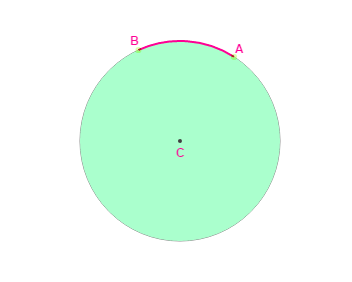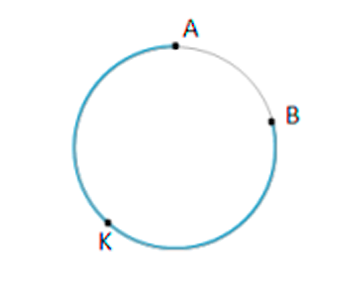# Arc

In Mathematics, an “arc” is a smooth curve joining two endpoints. In general, an arc is one of the portions of a circle. An arc can be a portion of some other curved shapes like an ellipse but mostly refers to a circle. In this article, let us discuss the arc of a circle, measures and arc length formula in a detailed way.

## Arc of a Circle

The arc of a circle is defined as the part or segment of the circumference of a circle.  A straight line that could be drawn by connecting the two ends of the arc is known as a chord of a circle. If the length of an arc is exactly half of the circle, it is known as a semicircular arc.An Arc is named based on its endpoints. The red arc in the above figure is called arc AB or BA since the order of points doesn’t matter. This can be expressed as the letter AB with a curved line above it, such as $\widehat{AB}$ and read as “arc AB”.

## Measures of an Arc

The arc can be measured using two different way. They are:

• The measure of a central angle
• The length of the arc

The arc’s length is computed in distance units, such as centimetres. To indicate it, the arc is preceded by the lowercase letter L(for ‘length’). For instance, $\widehat{lAB}$ = 7 inches is read as “the length of the arc AB is 7 inches”.### Arc Length Formula

The angle that is created by the arc at the middle of the circle is nothing but the angle measure. It’s described by the letter m preceding the name. For instance, $\widehat{mAB}$ = 600is read as “the arc AB has a measure of 60 degrees”.

Therefore, the arc length formula is given by:

When the central angle is measured in degrees, the arc length formula is:

Arc length = 2πr(θ/360)

where,

θ indicates the central angle of the arc in degrees

r indicates the radius of the arc

When the central angle is in radians, the arc length formula is:

Arc length = r. θ

Where,

θ indicates the central angle of the arc in radians.

r indicates the radius of the arc.

To know more about arc length of a sector and minor arc Math definition, register at BYJU’S – The Learning App.